## Audio

[Audio][slideshow]

## Differential amplifier

In this post, differential amplifier using BJT and differential amplifier using op-amps are explained in detail. Please go through both of them to get a better understanding.

• Differential Amplifier using Transistor
• Differential Amplifier using Op-amp

The circuit diagrams and detailed equations are provided along with the article. Please go through them.

Differential Amplifier using Transistor

A differential amplifier is designed to give the difference between two input signals. The circuit is shown below.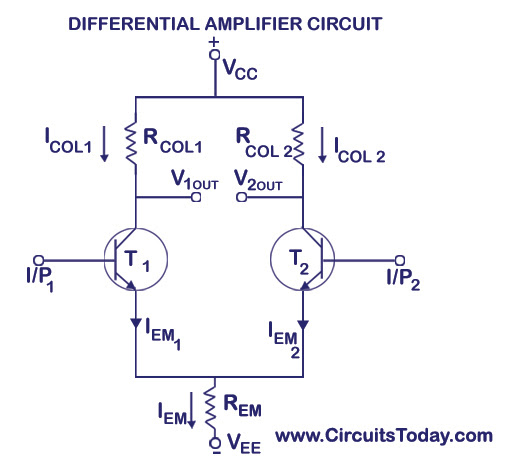Differential Amplifier Circuit

As shown in the circuit diagram above there are two inputs, I/P1 and I/P2 and two outputs V1OUT and V2OUT. I/P1 is applied to the base of the transistor TI and IP2 is applied to the base of the transistor T2. The emitters of both T1 and T2 are connected to a common emitter resistor so that the two output terminals V1OUT and V2OUT gets affected by the two input signals I/P1 and I/P2. VCC and VEE are the two supply voltages for the circuit. The circuit will also work fine using just a single voltage supply. You may have also noted that there is no ground terminal indicated in the circuit. Hence it must be automatically understood that the opposite points of both the positive and negative voltage supplies are understood to be connected to the ground.

### Working of a Differential Amplifier

When a differential amplifier is driven at one of the inputs, the output appears at both the collector outputs. This is explained with a diagram below.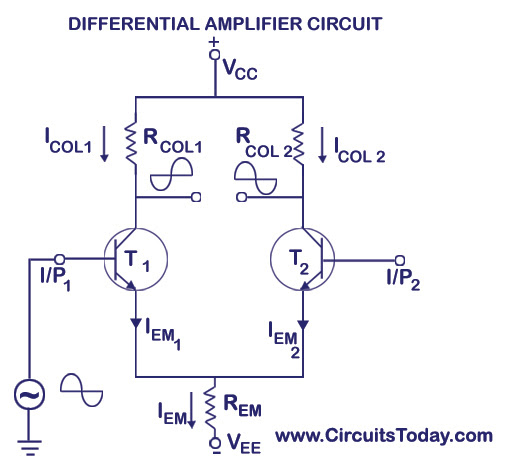Differential Amplifier Working

When input signal I/P1 is applied to the transistor T1, there will be a high voltage drop across the collector resistance RCOL1 , and thus the collector of T1 will be less positive. When I/P1 is negative T1 is turned OFF, and the voltage drop across RCOL1 becomes very low and thus the collector of T1 will be more positive. Thus we can conclude than an inserted output appears at T1’s collector for applying signal at I/P1.

When T1 is turned ON by the positive value of I/P1 , the current through the emitter resistance REM increases as the emitter current is almost equal to the collector current (IEIC). Thus the voltage drop across REM increases and makes the emitter of both transistors going in a positive direction. Making T2’s emitter positive is the same as making the base of T2 negative. In such a condition the transistor T2 will conduct less current which in turn will cause less voltage drop in RCOL2 and thus the collector of T2 will go in a positive direction for positive input signal. Thus we can conclude that the non-inverting output appears at the collector of transistor T2 for input at base of T1.

The amplification can be driven differentially by taking output between the collector of T1 and T2.

As shown in the figure above, if the transistor T1 and T2 are assumed to be identical in all characteristics, and if the voltages are equal (VBASE1 = VBASE2), then the emitter current can also be said to be eequal

IEM1 = IEM2

Total Emitter Current, IE = IEM1 + IEM2

VEM = VBASE – VBASE EM

IEM = (VBASE – VBASE EM)/REM

The emitter current IEM remains virtually constant regardless of the hfe value of the transistors.

Since ICOL1 IEM1, and ICOL2 IEM2, ICOL1 ICOL2

Also, VCOL1 = VCOL2 = VCC – ICOL RCOL, assuming collector resistance RCOL1 = RCOL2 = RCOL.

Differential amplifier is a closed loop amplifier circuit which amplifies the difference between two signals. Such a circuit is very useful in instrumentation systems. Differential amplifiers have high common mode rejection ratio (CMRR) and high input impedance. Differential amplifiers can be made using one opamp or two opamps. Both of these configurations are explained here.

### Differential Amplifier using Op-amp

The circuit diagram of a differential amplifier using one opamp is shown below. R1 and R2 are the input resistors, Rf is the feedback resistor and RL is the load resistor.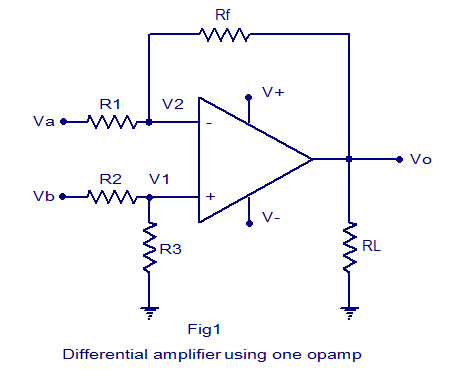Differential amplifier using one opamp

Derivation for voltage gain.

Equation for the voltage gain of the differential amplifier using one opamp can be derived as follows. The circuit is just a combination of an inverting and non inverting amplifier. Finding the output voltages s of these two configurations separately and then summing them will result in the overall output voltage.

If Vb is made zero, the circuit becomes an inverting amplifier. The output voltage Voa due to Va alone can be expressed using the following equation.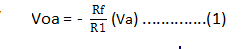When Va is made zero the circuit becomes a non inverting amplifier. Let V1 be the voltage at the non inverting input pin. Relation between Vb and V1 can be expressed using the following equation.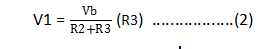Output voltage Vob due to Vb alone is according to the equation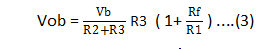Let R1 = R2 and R3 =Rf then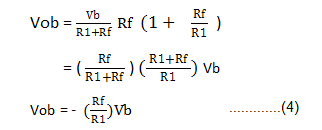Then overall output voltage isTherefore overall gain is

###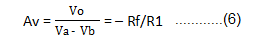Differential amplifier using two opamps.

Circuit diagram of a differential amplifier using two opamps is shown below. Main advantage of differential amplifier with two opamps is that it has increased overall gain. R1 is the input resistor for IC1 and R3 is the input resistor for IC2. Rf is the feedback resistor. Va and Vb are the two input voltages and they are applied to the non inverting inputs of IC2 and IC1 respectively. RL is the load resistor. V+ and V- are the positive and negative supply voltages.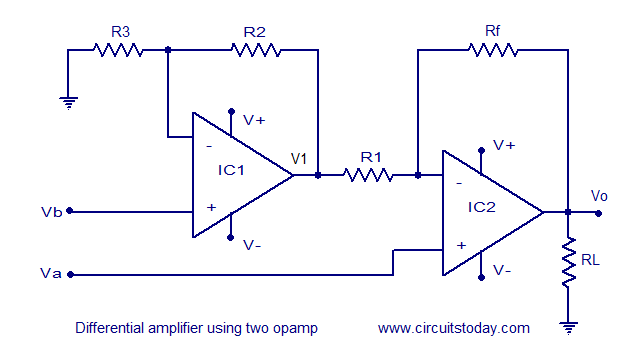Differential amplifier using opamp

Derivation of voltage gain.

The equation for the output voltage V1 of the first opamp (IC1) is as follows.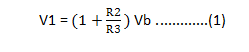V1 and Va are the inputs for the second stage (IC2). Output voltage due to Va alone is.Output voltage due to Vb alone is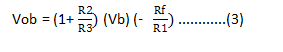Overall output voltage Vo = Voa + Vob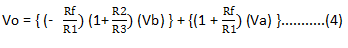Let R1 = R2 and Rf =R1, then we have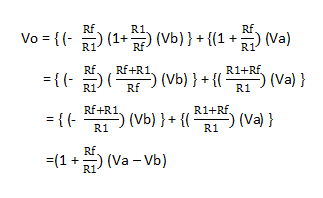Therefore overall voltage gain Av can be expressed using the equation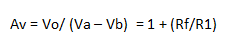### Practical differential amplifier.

A practical differential amplifier using uA741 opamp is shown below. With used components the amplifier has a gain of around 5. Remember the equation Av = -Rf/R1. Here Rf = 10K and R1 =2.2K, -Rf/R1 = -10/2.2 = -4.54 = ~-5. Negative sign represents phase inversion. Use +/-12V DC dual supply for powering the circuit. uA 741 must be mounted on a holder.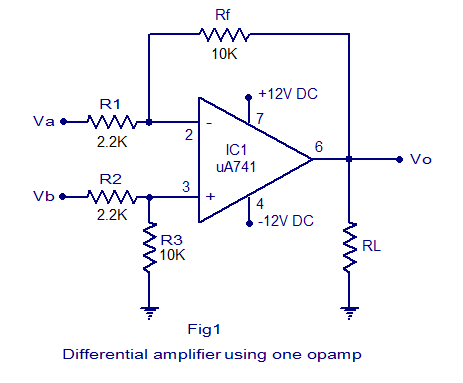Practical differential amplifier circuit

[Lights][grids]